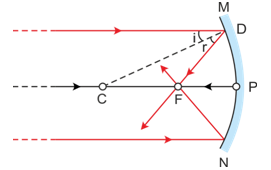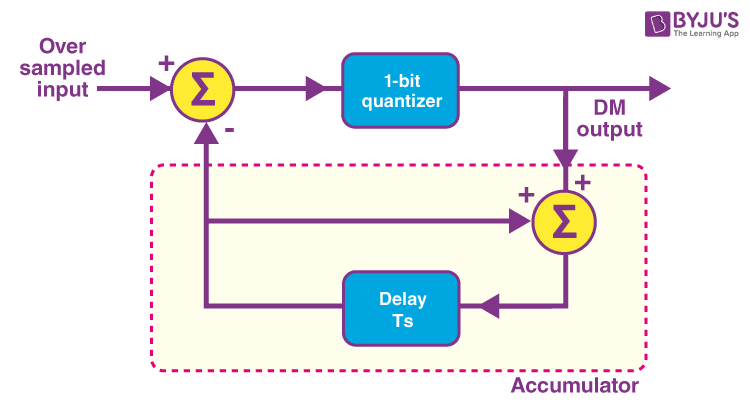Checkout JEE MAINS 2022 Question Paper Analysis : Checkout JEE MAINS 2022 Question Paper Analysis :

# Delta Modulation

In this article, let us study and understand a special form of digital modulation known as delta modulation.

## Delta Modulation

Nyquist rate or frequency is defined as the minimum rate at which a finite bandwidth signal needs to be sampled to retain all of the information. In order to get a comparatively better sampling rate in the differential pulse code modulation process, the signal’s sampling rate is maintained higher than the Nyquist rate.

In the Differential Pulse Code Modulation (DPCM) process, when the sampling interval is reduced, the sample-to-sample amplitude difference becomes small, like the difference is of 1-bit quantization. Hence the step-size will be very small.

Delta modulation is a process mainly used in the transmission of voice information. It is a technique where analog-to-digital and digital-to-analog signal conversion are seen. Delta modulation (DM) is an easy way of DPCM. In this technique, the difference between consecutive signal samples is encoded into n-bit data streams. In DM, the data which is to be transmitted is minimized to a 1-bit data stream.

## Block Diagram of Delta ModulatorThe sampling rate is comparatively very high in the delta modulation technique. The value of the step size after quantization is smaller. In the delta modulation process, the quantization design is easy and simple, and it gives the user the option to design the bit rate.

The delta modulator includes a 1-bit quantizer as shown in the figure above and a delay circuit along with two summer circuits. The output of the delta modulator will be a stair-case approximated waveform. The step size of this waveform is the delta (Δ). The output quality of the waveform is moderate. In order to obtain a high ratio signal-to-noise, DM must adapt oversampling techniques. In oversampling techniques, the analog signal is sampled many times higher than the Nyquist rate.

The bandwidth in bits/second is needed for the transmission of a delta-modulated signal. This signal is equal to the sampling frequency. We can find the bandwidth to transmit the modulated signal using the below formula.

Bandwidth required to transmit the modulated signal = ffs samples/second X 1 bit/sample

= ffs bits/second

Where,

fs is the signal’s sampling frequency

Delta Demodulator

Refer to the below figure for the delta demodulator. The delta demodulator includes a delay circuit, a low pass filter, and a summer connected as per the image below. Since the predictor circuit is removed, there is no assumed input given to the demodulator.A low pass filter is included in the circuit for noise elimination and to obtain better out-of-band signals. Granular noise is eliminated at the transmitter, and granular noise is referred to as the step-size error. When zero noise is seen, then the output of the modulator is equal to the demodulator input.

• Design is easy and simple.
• It is a 1-bit quantizer.
• Modulator & demodulator can be designed easily.
• In delta modulation, the quantisation design is very simple
• The bit rate can be designed by the user

• When the value of the delta is small, slope overload distortion is seen, which is a type of noise.
• When the value of delta is large, granular noise is seen, which is a type of noise.

An advanced form of delta modulation is adaptive delta modulation. As we know, granular noise and slope overload distortion are the types of noise seen in delta modulation. To eliminate these kinds of noise, an adaptive delta modulation technique was developed. Slope error seen in the delta modulation technique is reduced in this process. Slope overload error and granular error are completely removed using this process. Quantized noise is removed in the demodulation process using an LPF (low pass filter).

• Adaptive delta modulation offers extremely high performance.
• This technique decreases the need for correction circuits in radio design and error detection.
• Dynamic range is high since the variable step size covers a large range of values.
• Slope overload error and granular error are not seen.
• Slope error is reduced.

Stay tuned with BYJU’S for more such interesting articles on physics, chemistry and maths in an engaging way with video explanations.

## Frequently Asked Questions on Delta Modulation

Hertz.

Low pass filter.

### 3. In delta modulation, the transmitted data are reduced to?

1-bit data stream.

No.

Yes.

## Watch the video and learn the characteristics of sound waves.